Next: Exercises Up: Coupled Oscillations Previous: Two Coupled LC Circuits

# Three Spring-Coupled Masses

Consider a generalized version of the mechanical system discussed in Section 4.1 that consists of three identical masses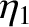which slide over a frictionless horizontal surface, and are connected by identical light horizontal springs of spring constant. As before, the outermost masses are attached to immovable walls by springs of spring constant. The instantaneous configuration of the system is specified by the horizontal displacements of the three masses from their equilibrium positions: namely,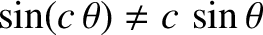,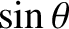, and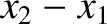. This is manifestly a three degree of freedom system. We, therefore, expect it to possess three independent normal modes of oscillation. Equations (149)-(150) generalize to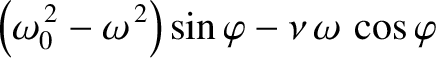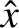(202)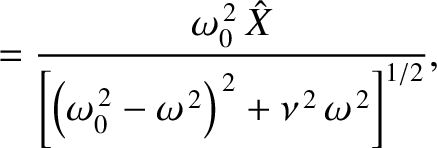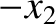(203)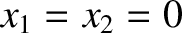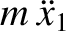(204)

These equations can be rewritten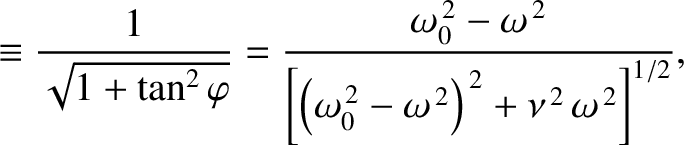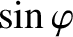(205)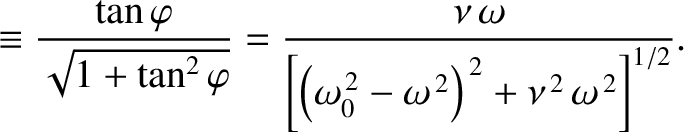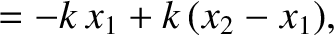(206)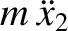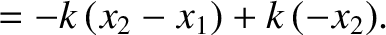(207)

where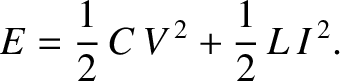. Let us search for a normal mode solution of the form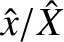(208)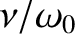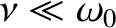(209)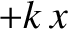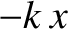(210)

Equations (205)-(210) can be combined to give the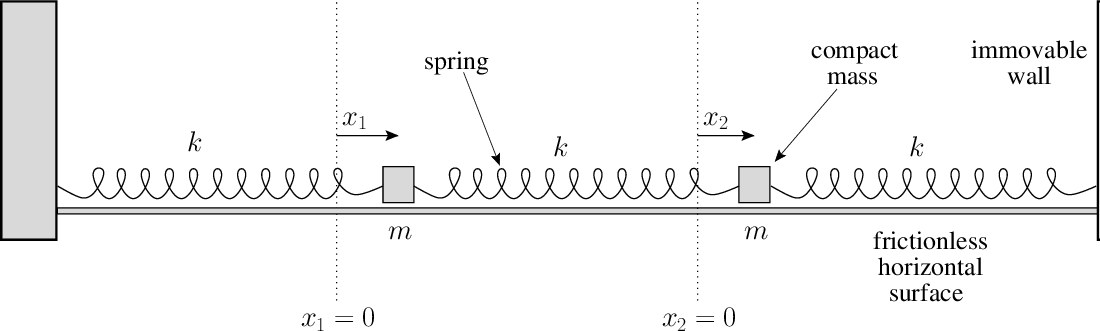homogeneous matrix equation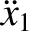(211)

where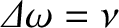. The normal frequencies are determined by setting the determinant of the matrix to zero: that is,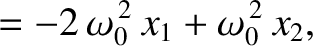(212)

or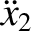(213)

Thus, the normal frequencies are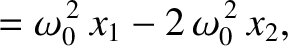,, and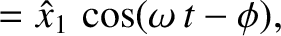. According to the first and third rows of Equation (211),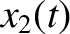(214)

provided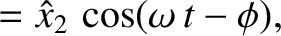. According to the second row,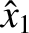(215)

when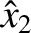. Incidentally, we can only determine the ratios of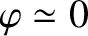,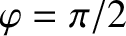, and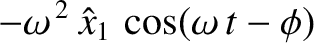, rather than the absolute values of these quantities. In other words, only the direction of the vector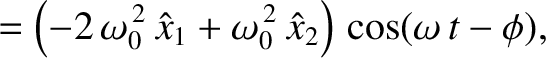is well-defined. [This follows because the most general solution, (219), is undetermined to an arbitrary multiplicative constant. That is, if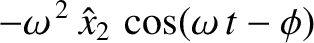is a solution to the dynamical equations (205)-(207) then so is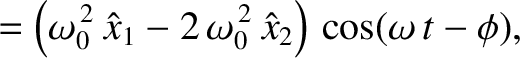, where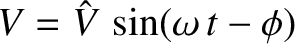is an arbitrary constant. This, in turn, follows because the dynamical equations are linear.] Let us arbitrarily set the magnitude of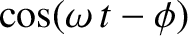to unity. It follows that the normal mode associated with the normal frequency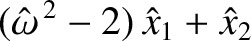is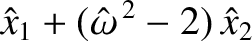(216)

Likewise, the normal mode associated with the normal frequency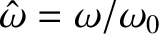is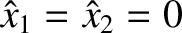(217)

Finally, the normal mode associated with the normal frequency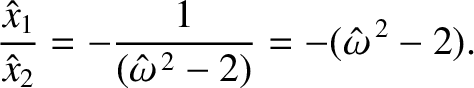is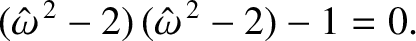(218)

The vectors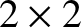,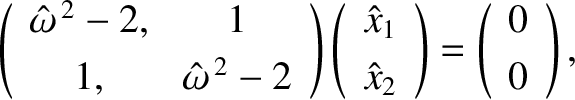, and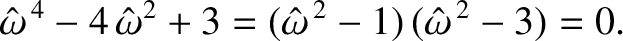are mutually perpendicular. In other words, they are normal vectors. (Hence, the name normal'' mode.)

Let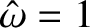. It follows that the most general solution to the problem is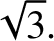(219)

where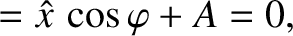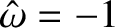(220)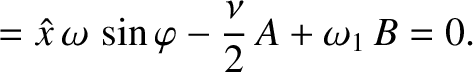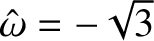(221)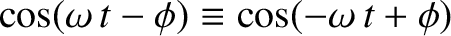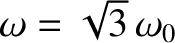(222)

Here,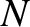andare constants. Incidentally, we need to introduce the arbitrary amplitudesto make up for the fact that we set the magnitudes of the vectors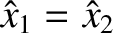to unity. Equation (219) yields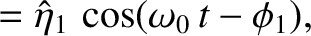(223)

The preceding equation can be inverted by noting that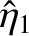, et cetera, because,, andare mutually perpendicular unit vectors. Thus, we obtain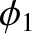(224)

This equation determines the three normal coordinates,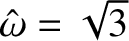,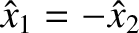,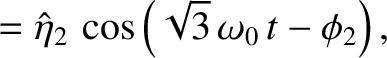, in terms of the three conventional coordinates,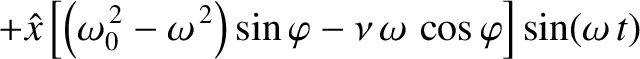,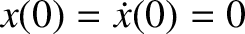,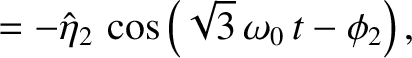. In general, the normal coordinates are undetermined to arbitrary multiplicative constants.Next: Exercises Up: Coupled Oscillations Previous: Two Coupled LC Circuits
Richard Fitzpatrick 2013-04-08